# LDA: Latent Dirichlet Allocation

## Motivation

Bag-of-words models in general performs quite well, and we're looking for a probabilistic model of text. Let's look at the underlying assumptions of a bag-of-word model:

• Ordering of words within a document is irrelevant
• Ordering of documents in entire corpus is irrelevant

## Notation

• word is the basic unit of discrete data, defined to be an item from a vocabulary indexed by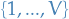. Represented using unit-basis vectors that have a single component equal to 1 and all other components equal to 0. Thus, using superscripts to denote components,the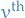word in the vocabulary is represented by a V-vector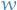such that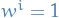and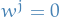for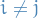.
• document is a sequence of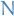words denoted by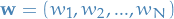where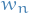is the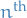word in the sequence
• corpus is a collection of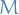documents denoted by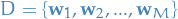.
• topic is characterized by a distribution over words

## Latent Dirichlet Allocation

• Generative model of a corpus
• Documents are represented as a random mixtures over latent topics

### Notation

•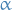parameter for Dirichlet distribution
•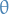latent random variable for some document (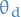for a specific document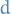) drawn from a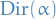•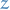is a k-dimensional one-hot-vector representing the chosen topic for a word i.e. with the i-th component equal to 1 and all other components being 0 (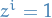for a unique)
•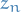is the same as, but for the specific n-th wordin a document, i.e. topic for this word.
•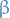is a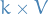matrix parameterizing the word probabilities, i.e.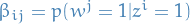### Model

Assume the following generative model for each document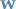in a corpus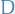:

1. Choose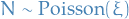, i.e. the length of the document (following this distribution is not a strict requirement)
2. Choose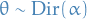, used as a parameter for a multinomial later on
3. Each wordin the document of lengthis assigned as follows:
1. Choose a topic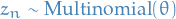2. Choose a word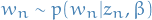, multinomial distribution conditioned on the topic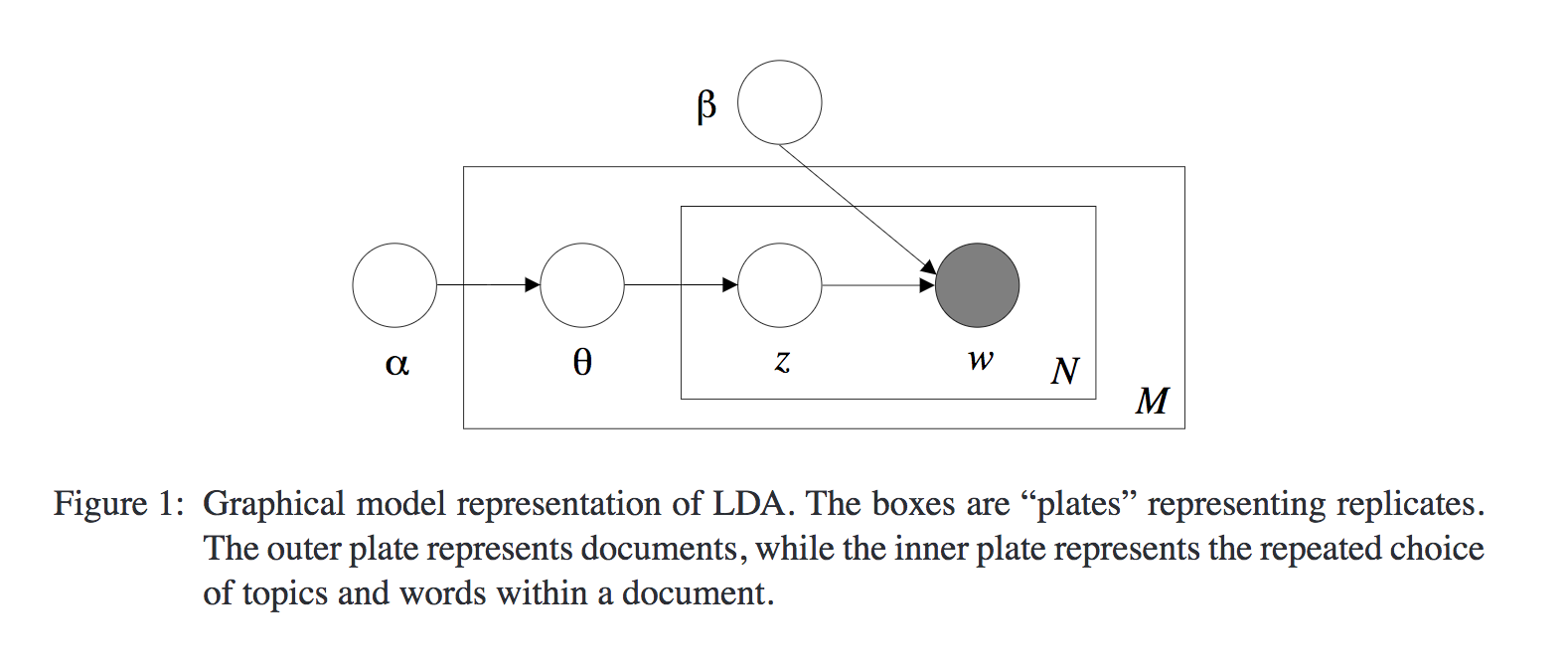#### Dirichlet

##### My interpretation

From a Dirichlet we draw a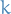-vector which corresponds to a multinomial distribution, with each component corresponding to the probability of the corresponding topic / label / class. In our case,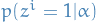then corresponds to the i-th component of, i.e. the probability of topic.

##### From the paper

A k-dimensional Dirichlet random variablecan take values in the (k−1)-simplex (a k-vectorlies in the (k − 1)-simplex if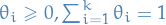), and has the following probability density on this simplex: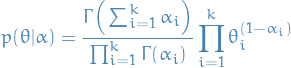1

When we're talking about the (k - 1)-simplex we're referring to a region of space where the parameters of the mutlinomial can be (domain of all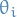). Why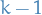? Since we require that the sum over allequals 1, after choosing a value forof these, the last one is determined as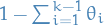.

Theandbeing referred to in this section on the Dirichlet has nothing to do with notation used elswhere in this text, and simply represents some arbitrary integerand some random variable.

#### Joint distributions

First we have the joint distribution over the topic mixture, topics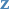and wordsfor one document: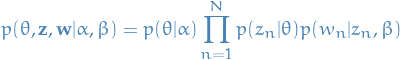2

Where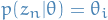where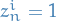sinceis a one-hot vector representing the n-th chosen topic.

topic mixture simply refers to a latent variable which the rv. topic depends on.

Then to obtain the probability of a document, i.e. a set of words, we simply integrate over all topic mixturesand summing over all possible topic assignments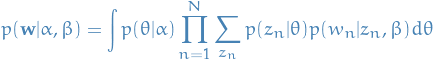3

And finally, to obtain the distribution over our corpus, i.e. our set of documents, we simply take the product over all documents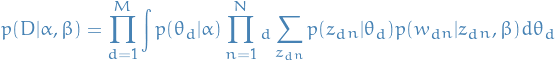4

#### Inference

Our inference problem is to compute the distributions of the latent variablesandgiven a document.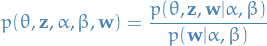5

Both of which we deduced in the previous section! Unfortunately, this computation is intractable. Luckily there exists methods to deal with this: variational inference, MCMC and Laplace approximation.